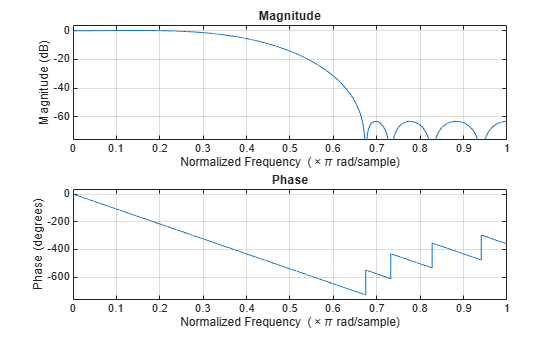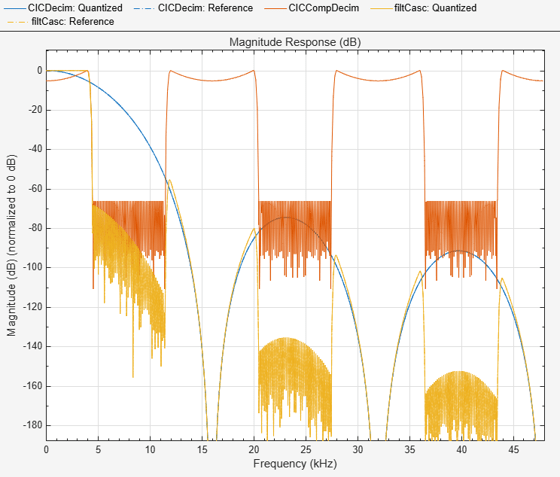# dsp.CICCompensationDecimator

Compensate for CIC decimation filter using FIR decimator

## Description

You can compensate for the shortcomings of a CIC decimator, namely its passband droop and wide transition region, by following it with a compensation decimator. This System object™ lets you design and use such a filter.

To compensate for the shortcomings of a CIC filter using an FIR decimator:

1. Create the `dsp.CICCompensationDecimator` object and set its properties.

2. Call the object with arguments, as if it were a function.

## Creation

### Syntax

``ciccompdec = dsp.CICCompensationDecimator``
``ciccompdec = dsp.CICCompensationDecimator(decim)``
``ciccompdec = dsp.CICCompensationDecimator(cic)``
``ciccompdec = dsp.CICCompensationDecimator(cic,decim)``
``ciccompdec = dsp.CICCompensationDecimator(___,Name,Value)``

### Description

````ciccompdec = dsp.CICCompensationDecimator` returns a System object, `ciccompdec`, that applies an FIR decimator to each channel of an input signal. Using the properties of the object, the decimation filter can be designed to compensate for a preceding CIC filter.```
````ciccompdec = dsp.CICCompensationDecimator(decim)` returns a CIC compensation decimator System object, with the `DecimationFactor` property set to `decim`.```
````ciccompdec = dsp.CICCompensationDecimator(cic)` returns a CIC compensation decimator System object, with the `CICRateChangeFactor`, `CICNumSections`, and `CICDifferentialDelay` properties specified in the `dsp.CICDecimator` System object, `cic`.```
````ciccompdec = dsp.CICCompensationDecimator(cic,decim)` returns a CIC compensation decimator System object, `ciccompdec`, with the `CICRateChangeFactor`, `CICNumSections`, and `CICDifferentialDelay` properties specified in the `dsp.CICDecimator` System object `cic`, and the `DecimationFactor` property set to `decim`.```

example

````ciccompdec = dsp.CICCompensationDecimator(___,Name,Value)` returns a CIC compensation decimator object with each specified property set to the specified value. Enclose each property name in quotes. You can use this syntax with any previous input argument combinations.```

## Properties

expand all

Unless otherwise indicated, properties are nontunable, which means you cannot change their values after calling the object. Objects lock when you call them, and the `release` function unlocks them.

If a property is tunable, you can change its value at any time.

Specify the differential delay of the CIC filter being compensated as a positive integer scalar.

Data Types: `single` | `double` | `int8` | `int16` | `int32` | `int64` | `uint8` | `uint16` | `uint32` | `uint64`

Specify the number of sections of the CIC filter being compensated as a positive integer scalar.

Data Types: `single` | `double` | `int8` | `int16` | `int32` | `int64` | `uint8` | `uint16` | `uint32` | `uint64`

Specify the rate-change factor of the CIC filter being compensated as a positive integer scalar.

Data Types: `single` | `double` | `int8` | `int16` | `int32` | `int64` | `uint8` | `uint16` | `uint32` | `uint64`

Specify the decimation factor of the compensator System object as a positive integer scalar.

Data Types: `single` | `double` | `int8` | `int16` | `int32` | `int64` | `uint8` | `uint16` | `uint32` | `uint64`

Specify whether to design a filter of minimum order or a filter of specified order as a logical scalar. The default is `true`, which corresponds to a filter of minimum order.

Specify the order of the decimation compensator filter as a positive integer scalar.

#### Dependencies

This property applies only when you set the `DesignForMinimumOrder` property to `false`.

Data Types: `single` | `double` | `int8` | `int16` | `int32` | `int64` | `uint8` | `uint16` | `uint32` | `uint64`

Specify the passband edge frequency as a positive real scalar expressed in hertz. `PassbandFrequency` must be less than Fs/2, where Fs is the input sample rate.

Data Types: `single` | `double` | `int8` | `int16` | `int32` | `int64` | `uint8` | `uint16` | `uint32` | `uint64`

Specify the filter passband ripple as a positive real scalar expressed in decibels.

Data Types: `single` | `double` | `int8` | `int16` | `int32` | `int64` | `uint8` | `uint16` | `uint32` | `uint64`

Specify the input sample rate as a positive real scalar expressed in hertz.

Data Types: `single` | `double`

Specify the filter stopband attenuation as a positive real scalar expressed in decibels.

Data Types: `single` | `double` | `int8` | `int16` | `int32` | `int64` | `uint8` | `uint16` | `uint32` | `uint64`

Specify the stopband edge frequency as a positive real scalar expressed in hertz. `StopbandFrequency` must be less than Fs/2, where Fs is the input sample rate.

Data Types: `single` | `double` | `int8` | `int16` | `int32` | `int64` | `uint8` | `uint16` | `uint32` | `uint64`

### Fixed-Point Properties

Word and fraction lengths of coefficients, specified as a signed or unsigned `numerictype` object. The default, `numerictype(1,16)` corresponds to a signed numeric type object with 16-bit coefficients and a fraction length determined based on the coefficient values, to give the best possible precision.

This property is not tunable.

Word length of the output is same as the word length of the input. Fraction length of the output is computed such that the entire dynamic range of the output can be represented without overflow. For details on how the fraction length of the output is computed, see Fixed-Point Precision Rules for Avoiding Overflow in FIR Filters.

Rounding method for output fixed-point operations, specified as a character vector. For more information on the rounding modes, see Precision and Range.

## Usage

### Syntax

``y = ciccompdecim(x)``

### Description

example

````y = ciccompdecim(x)` returns the filtered and downsampled values, `y`, of the input signal, `x`. ```

### Input Arguments

expand all

Data input, specified as a vector or a matrix. The System object treats a Ki × N input matrix as N independent channels, decimating each channel over the first dimension.

This object does not support complex unsigned fixed-point data.

Data Types: `single` | `double` | `int8` | `int16` | `int32` | `int64` | `uint8` | `uint16` | `uint32` | `uint64` | `fi`
Complex Number Support: Yes

### Output Arguments

expand all

Filtered and downsampled signal, returned as a vector or matrix. For a Ki × N input matrix, the result is a Ko × N output matrix, where Ko = Ki / M and M is the decimation factor.

Data Types: `single` | `double` | `int8` | `int16` | `int32` | `int64` | `uint8` | `uint16` | `uint32` | `uint64` | `fi`
Complex Number Support: Yes

## Object Functions

To use an object function, specify the System object as the first input argument. For example, to release system resources of a System object named `obj`, use this syntax:

`release(obj)`

expand all

 `freqz` Frequency response of filter `fvtool` Visualize frequency response of DSP filters `info` Information about filter System object `cost` Estimate cost of implementing filter System objects `coeffs` Filter coefficients `polyphase` Polyphase decomposition of multirate filter `generatehdl` Generate HDL code for quantized DSP filter (requires Filter Design HDL Coder)
 `step` Run System object algorithm `release` Release resources and allow changes to System object property values and input characteristics `reset` Reset internal states of System object

## Examples

collapse all

Design an CIC compensation decimator. Specify the decimation factor to be 2, passband frequency to be 4 kHz, stopband frequency to be 4.5 kHz, and the input sample rate to be 16 kHz.

```fs = 16e3; fPass = 4e3; fStop = 4.5e3; CICCompDecim = dsp.CICCompensationDecimator('DecimationFactor',2,'PassbandFrequency',fPass, ... 'StopbandFrequency',fStop,'SampleRate',fs);```

Plot the impulse response. The group delay of the filter is 45.5.

`fvtool(CICCompDecim,'Analysis','impulse')`Plot the magnitude and Phase response.

`fvtool(CICCompDecim,'Analysis','freq')`Note: If you are using R2016a or an earlier release, replace each call to the object with the equivalent step syntax. For example, `obj(x)` becomes `step(obj,x)`.

Design a compensation decimator for an existing CIC decimator having six sections and a decimation factor of 6.

```CICDecim = dsp.CICDecimator('DecimationFactor',6, ... 'NumSections',6);```

Construct the compensation decimator. Specify a decimation factor of 2, an input sample rate of 16 kHz, a passband frequency of 4 kHz, and a stopband frequency of 4.5 kHz.

```fs = 16e3; fPass = 4e3; fStop = 4.5e3; CICCompDecim = dsp.CICCompensationDecimator(CICDecim, ... 'DecimationFactor',2,'PassbandFrequency',fPass, ... 'StopbandFrequency',fStop,'SampleRate',fs);```

Visualize the frequency response of the cascade. Normalize all magnitude responses to 0 dB.

```filtCasc = dsp.FilterCascade(CICDecim,CICCompDecim); f = fvtool(CICDecim, CICCompDecim, filtCasc, ... 'Fs', [fs*6 fs fs*6]); f.NormalizeMagnitudeto1 = 'on'; legend(f,'CIC Decimator','CIC Compensation Decimator', ... 'Overall Response');```Apply the design to a 1200-sample random input signal. Store the decimated output along the first dimension of the y array.

```x = dsp.SignalSource(fi(rand(1200,1),1,16,15),'SamplesPerFrame',120); y = fi(zeros(100,1),1,32,20); for ind = 1:10 x2 = CICDecim(x()); y(((ind-1)*10)+1:ind*10,1) = CICCompDecim(x2); end```

## Algorithms

The response of a CIC filter is given by:

`${H}_{cic}\left(\omega \right)={\left[\frac{\mathrm{sin}\left(\frac{RD\omega }{2}\right)}{\mathrm{sin}\left(\frac{\omega }{2}\right)}\right]}^{N}$`

R, D, and N are the rate change factor, the differential delay, and the number of sections of the CIC filter, respectively.

After decimation, the cic response has the form:okay

`${H}_{cic}\left(\omega \right)={\left[\frac{\mathrm{sin}\left(\frac{D\omega }{2}\right)}{\mathrm{sin}\left(\frac{\omega }{2R}\right)}\right]}^{N}$`

The normalized version of this last response is the one that the CIC compensator needs to compensate. Hence, the passband response of the CIC compensator should take the following form:

`${H}_{ciccomp}\left(\omega \right)={\left[RD\frac{\mathrm{sin}\left(\frac{\omega }{2R}\right)}{\mathrm{sin}\left(\frac{D\omega }{2}\right)}\right]}^{N}\text{for}\text{\hspace{0.17em}}\omega \le {\omega }_{p}<\pi$`

where ωp is the passband frequency of the CIC compensation filter.

Notice that when ω/2R ≪ π, the previous equation for Hciccomp(ω) can be simplified using the fact that sin(x) ≅ x:

This previous equation is the inverse sinc approximation to the true inverse passband response of the CIC filter.

Watch now